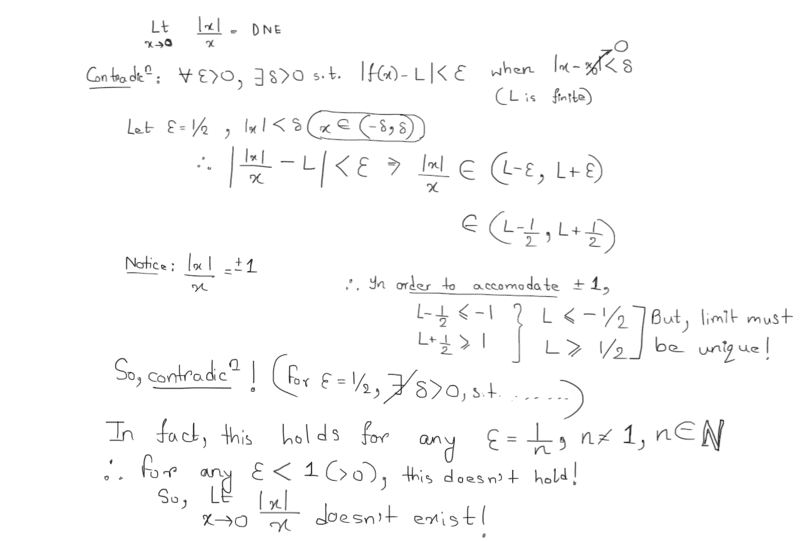# Prove that the limit of |x|/x at x=0 DNE

Homework Statement:
.
Relevant Equations:
.
Problem: Prove that $$\lim_{x\to 0}|\frac{|x|} x=\text{Undefined}$$

The solution written by my prof. uses a special case where the tolerance of error ##\epsilon=1/2##. However, I want to proof it with generality, meaning that the tolerance is shrinking.

Below is my attempt at a solution:
$$0<|x-0|<\delta;\, |f(x)-L|<\epsilon,\,\forall \epsilon>0$$
$$0<x^2<\delta^2;\, (f(0)-L)^2<\epsilon^2$$
$$\begin{cases} f(x>0)=1\\ f(x<0)=-1 \end{cases}$$
This is where I am stuck. It looks the two part of the function are independent of ##\delta##, making it impossible to establish a relationship with ##\epsilon##. Can somebody please help?

Last edited:

FactChecker
Gold Member
The solution written by my prof. uses a special case where the tolerance of error ##\epsilon=1/2##. However, I want to proof it with generality, meaning that the tolerance is shrinking.
Consider carefully what your professor's proof is doing. It says that there are points where the function values stay away from any proposed limit by at least 1/2. Doesn't that also work for any ##\epsilon \lt 1/2##?

•Leo Liu
Consider carefully what your professor's proof is doing. It says that there are points where the function values stay away from any proposed limit by at least 1/2. Doesn't that also work for any ##\epsilon \lt 1/2##?
She discusses the two parts separately and proves the limit DNE by arguing that there are two possible disjoint ranges in which L may lie.

Delta2
Homework Helper
Gold Member
The only other way to do this that I can see is to consider the limits ##\lim_{x\to 0^{+}}f(x)## and ##\lim_{x\to 0^{-}}f(x)## and since they are not equal the limit does not exist, because if it existed they would be equal. I am afraid your professor's way is the only way using epsilon delta proof, the only thing that could change is the value of epsilon.

••Demystifier, PhysicsTruth, PeroK and 1 other person
PeroK
Homework Helper
Gold Member
2020 Award
Homework Statement:: .
Relevant Equations:: .

Problem: Prove that $$\lim_{x\to 0}|\frac{|x|} x=\text{Undefined}$$

The solution written by my prof. uses a special case where the tolerance of error ##\epsilon=1/2##. However, I want to proof it with generality, meaning that the tolerance is shrinking.

Below is my attempt at a solution:
$$0<|x-0|<\delta;\, |f(x)-L|<\epsilon,\,\forall \epsilon>0$$
$$0<x^2<\delta^2;\, (f(0)-L)^2<\epsilon^2$$
$$\begin{cases} f(x>0)=1\\ f(x<0)=-1 \end{cases}$$
This is where I am stuck. It looks the two part of the function are independent of ##\delta##, making it impossible to establish a relationship with ##\epsilon##. Can somebody please help?
This is not mathematics. All I see are disconnected statements with no logical flow.

••Delta2 and Leo LiuIn order to prove the existence of a limit, the underlying condition is that for every ##\epsilon >0##, there exists ##\delta >0##. If you can show that this doesn't hold for a single value of ##\epsilon##, you are done. Also, you can observe that any ##\epsilon <1## works here, as the two sets of relations you can produce are always disjoint in that case.

••Leo Liu and Delta2
PeroK
Homework Helper
Gold Member
2020 Award
This is where the sequential definition of a limit is useful:
$$\lim_{x \rightarrow a}f(x) = L$$ iff for every sequence ##\{x_n\}## in ##\mathbb{R}-\{a\}## we have $$\lim_{n \rightarrow \infty} x_n = a \ \Rightarrow \ \lim_{n \rightarrow \infty} f(x_n) = L$$
It's a good exercise to show this is equivalent to the ##\epsilon-\delta## definition.

In this case, you show that positive and negative sequences lead to different limits for the function values. This is similar to calculating the one-sided limits, as suggested above. But, in general, this sequential definition is more flexible (e.g. when you have things involving rationals and irrationals).

•PhysicsTruth
anuttarasammyak
Gold Member
Say we note the function as f,
x ##\rightarrow## +0 f##\rightarrow## 1
x ##\rightarrow## -0 f##\rightarrow## -1
So
x ##\rightarrow## 0 f has no limit value.
f(x)=2H(x)-1
where H is Heaviside step function which has parameter of value H(0) as we like. Cf. https://en.wikipedia.org/wiki/Heaviside_step_function

•Leo Liu
PeroK
Homework Helper
Gold Member
2020 Award
This is not mathematics. All I see are disconnected statements with no logical flow.
@Leo Liu If, for example, you are trying to show that a limit does not exist, then technically you need the negation of the limit. In this case:
$$\exists \epsilon > 0: \ \forall \delta > 0, \exists x: 0 < |x - a| < \delta \ \text{and} \ |f(x) - L| \ge \epsilon$$
This, however, says that a particular ##L## is not the limit. What we really want is to show that for all ##L \in \mathbb R##. Just for fun, let's do that anyway!:
$$\forall L \in \mathbb R: \exists \epsilon > 0: \ \forall \delta > 0, \exists x: 0 < |x - a| < \delta \ \text{and} \ |f(x) - L| \ge \epsilon$$
When you look at it like that it's no wonder you can get tangled up in the logic of showing formally that the limit does not exist. That's another reason to prefer the sequential approach or the one-sided limits. And, in general, it's a good idea to look for theorems that simplify what you need to show.

In any case, it's really important to have a logical flow to a proof and be crystal clear about what you are doing ar every step.

•Delta2 and Leo Liu
Thank you all!

•Delta2